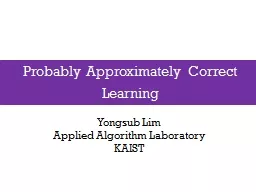# Probably Approximately Correct Learning2016-05-22 63K 63 0 0

## Probably Approximately Correct Learning - Description

Yongsub. Lim. Applied Algorithm Laboratory. KAIST. Definition. A class is . PAC learnable . by a hypothesis class if. there is an algorithm such that. over . ID: 329642 Download Presentation

Embed code:

## Probably Approximately Correct Learning

Download Presentation - The PPT/PDF document "Probably Approximately Correct Learning" is the property of its rightful owner. Permission is granted to download and print the materials on this web site for personal, non-commercial use only, and to display it on your personal computer provided you do not modify the materials and that you retain all copyright notices contained in the materials. By downloading content from our website, you accept the terms of this agreement.

### Presentations text content in Probably Approximately Correct Learning

Slide1

Probably Approximately Correct Learning

Yongsub

Lim

Applied Algorithm Laboratory

KAIST

Slide2

Definition

A class is PAC learnable by a hypothesis class ifthere is an algorithm such that over , # of i.i.d. training examples sampled from , such that where is an output of

Probably Approximately Correct Learning

2

Slide3

Example

Consider the class space which is the set of all positive half-linesAn example is any real numberEg)

Probably Approximately Correct Learning

1

0

3

Slide4

Proof. is PAC learnable

is PAC learnable by

Probably Approximately Correct Learning

4

Slide5

Proof. is PAC learnable

Our algorithm outputs a hypothesis such that

Suppose for a positive example for a negative example

Probably Approximately Correct Learning

5

Slide6

Proof. is PAC learnable

Suppose , and it called

only occurs if no training example

Probably Approximately Correct Learning

6

Slide7

Proof. is PAC learnable

Probably Approximately Correct Learning

7

Slide8

Proof. is PAC learnable

Probably Approximately Correct Learning

8

Slide9

Proof. is PAC learnable

The class is PAC learnable by itself with at least training examples

Probably Approximately Correct Learning

9

Slide10

A More General Theorem

Probably Approximately Correct Learning

10

Slide11

Thanks

Probably Approximately Correct Learning

11

Slide12

Slide13

Slide14

Slide15